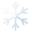# Potion

This item is of drink class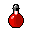You see a potion. Take it to battle and you may survive longer.
Attributes
AMOUNT
100
FREQUENCY
1
REGEN
100
Drink|Use
Dropped by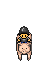cavalryman gnome
Drops exactly 1.
Probability: 20%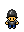infantry gnome
Drops exactly 1.
Probability: 20%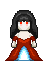vampire bride
Drops between 1 and 2.
Probability: 30%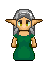mage elf
Drops between 2 and 4.
Probability: 20%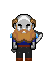duergar axeman
Drops between 1 and 2.
Probability: 1.5%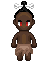elder cannibal
Drops between 1 and 3.
Probability: 20%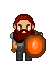leader dwarf
Drops between 1 and 2.
Probability: 1.5%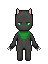cat woman
Drops between 1 and 3.
Probability: 10%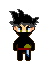disciple assassin
Drops exactly 1.
Probability: 10.5%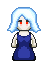vampirette
Drops between 1 and 2.
Probability: 30%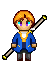imperial priest
Drops between 1 and 2.
Probability: 10%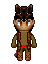barbarian wolf
Drops between 1 and 2.
Probability: 30%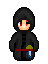apprentice assassin
Drops exactly 1.
Probability: 15.5%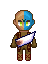barbarian elite
Drops between 1 and 2.
Probability: 30%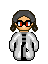imperial scientist
Drops between 2 and 4.
Probability: 8%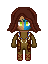barbarian priest
Drops between 1 and 2.
Probability: 30%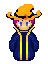imperial high priest
Drops between 1 and 2.
Probability: 10%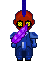blue mohiko
Drops between 1 and 2.
Probability: 10%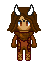barbarian chaman
Drops between 1 and 2.
Probability: 30%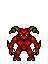imp
Drops between 1 and 2.
Probability: 30%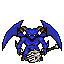winged blue imp
Drops between 1 and 2.
Probability: 30%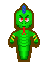high naga
Drops between 1 and 2.
Probability: 30%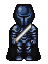chaos warrior
Drops between 1 and 2.
Probability: 30%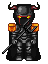chaos dragonrider
Drops between 1 and 2.
Probability: 30%